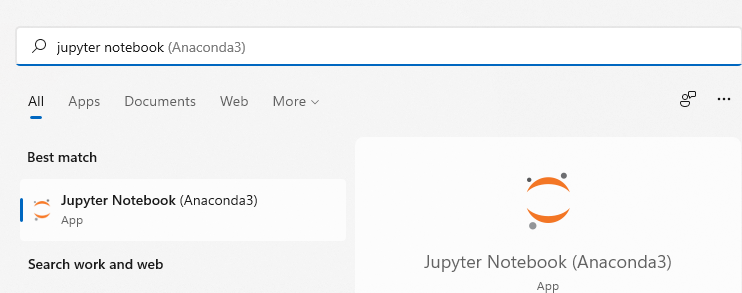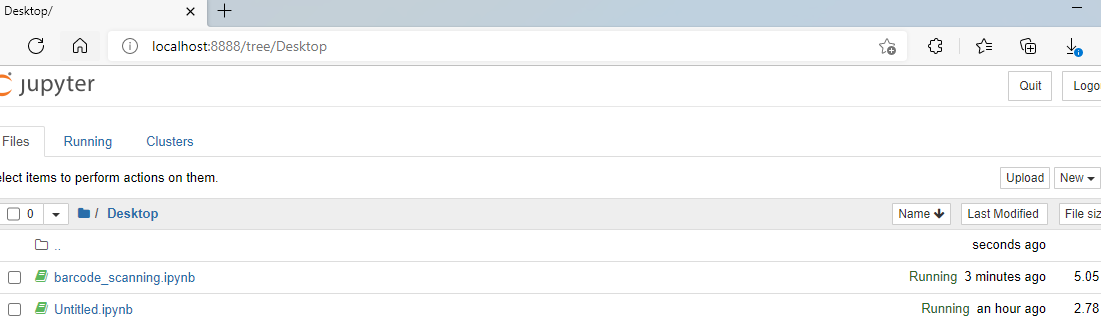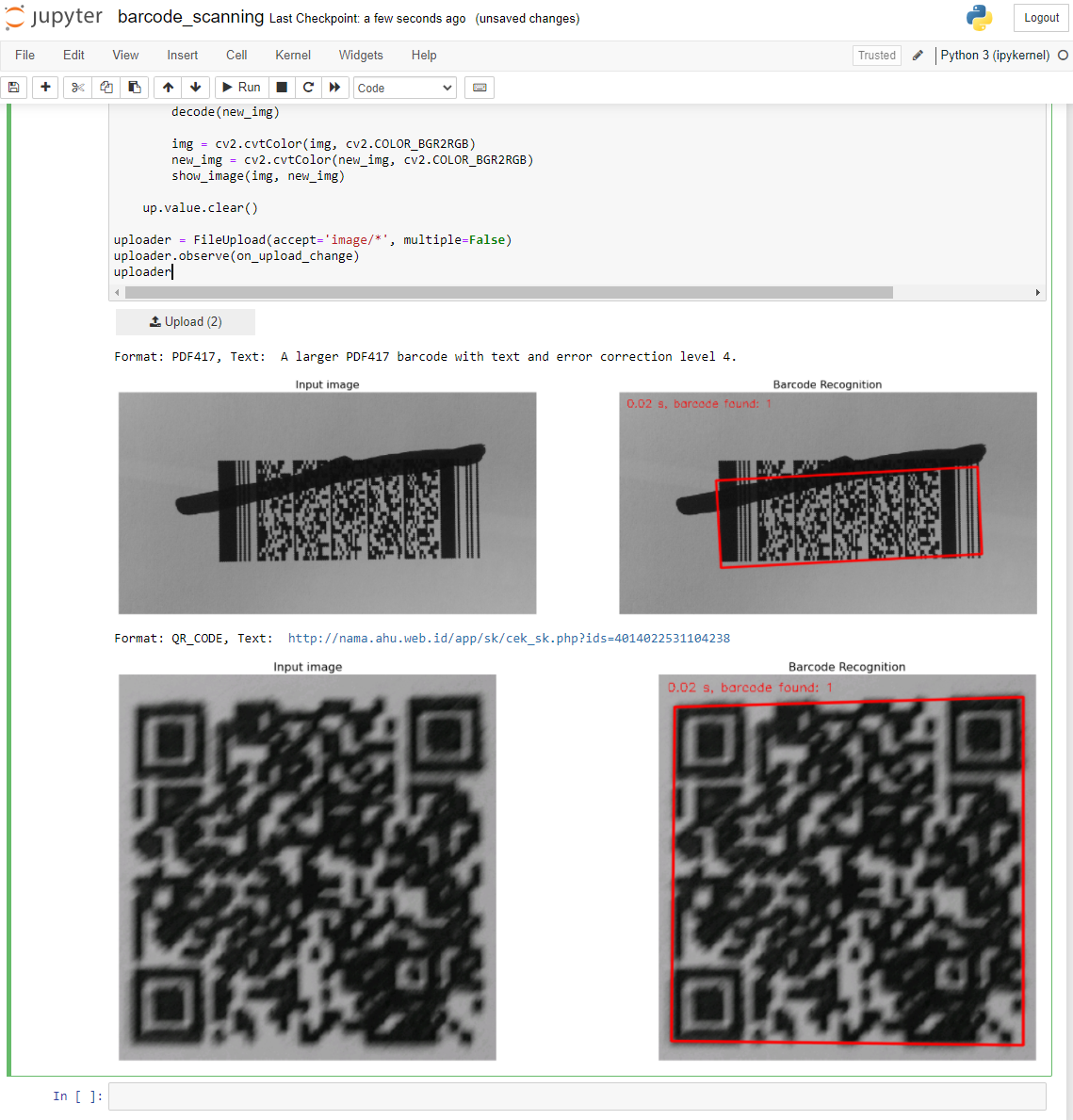# How to Scan Barcodes in Jupyter Notebook

Jupyter notebook is a web-based interactive computing environment that enables you to create and share documents that contain live code, equations, visualizations and narrative text. If you have installed Anaconda, Jupyter notebook is installed by default. This article demonstrates how to write Python code to scan barcodes in Jupyter notebook.

## Creating a Jupyter Notebook for Python Barcode Scanning

1. Run Jupyter notebook:2. Open `http://localhost:8888/` in your browser to create a `barcode_scanning.ipynb` file.3. Insert a new cell to install Dynamsoft Barcode Reader, OpenCV, and Matplotlib:

`````` !pip install dbr opencv-python matplotlib
``````

`````` from dbr import *
``````
5. Create an image upload button in Jupyter notebook:

`````` from ipywidgets import FileUpload
if not change.new:
return
up = change.owner

up.value.clear()

``````
6. Convert the image data to numpy array and then decode the image with OpenCV API:

`````` import cv2 as cv
import numpy as np

up = change.owner
for _, data in up.value.items():
image_buffer = np.frombuffer(data['content'], dtype=np.uint8)
img = cv.imdecode(image_buffer, 1)

up.value.clear()
``````
7. Scan barcodes with Dynamsoft Barcode API:

`````` def decode(frame):

before = time.time()
after = time.time()

COLOR_RED = (0,0,255)
thickness = 2
margin = 1
text_x = 10; text_y = 20
if results != None:
found = len(results)
for result in results:
print("Format: %s, Text: %s" % (result.barcode_format_string, result.barcode_text))
text = result.barcode_text
points = result.localization_result.localization_points
data = np.array([[points, points], [points, points], [points, points], [points, points]])
cv.drawContours(image=frame, contours=[data], contourIdx=-1, color=COLOR_RED, thickness=thickness, lineType=cv.LINE_AA)

cv.putText(frame, '%.2f s, barcode found: %d' % (after - before, found), (text_x, text_y), cv.FONT_HERSHEY_SIMPLEX, 0.5, COLOR_RED)
else:
cv.putText(frame, '%.2f s, barcode found: %d' % (after - before, 0), (text_x, text_y), cv.FONT_HERSHEY_SIMPLEX, 0.5, COLOR_RED)

img = cv.imdecode(image_buffer, 1)

new_img = img.copy()
# barcode recognition
decode(new_img)
``````
8. Display the barcode recognition results with Matplotlib:

`````` import matplotlib.pyplot as plt

def show_image(img1, img2):
fig = plt.figure(figsize=(25, 10))
plt.title('Input image', fontsize=16)
ax1.axis('off')
plt.title('Barcode Recognition', fontsize=16)
ax2.axis('off')
ax1.imshow(img1)
ax2.imshow(img2)

img = cv.cvtColor(img, cv.COLOR_BGR2RGB)
new_img = cv.cvtColor(new_img, cv.COLOR_BGR2RGB)
show_image(img, new_img)
``````So far, the Jupyter notebook is done. To make it accessible to other users, we can share it via Google Drive.## Source Code

https://github.com/yushulx/jupyter-notebook-barcode-scanning